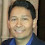# ANGULARJS Calculate Totals Using Directives

In this article, we show how to do a calculation of subtotals and totals using AngularJS. To illustrate how we can do the aggregates, we build a simple invoice in which we will add the total quantity of items and subtotals per item and final amount.

We first start by showing the JSON model that represents a list of items with quantity and cost per unit. These are the amounts that we will be adding using directives.

 \$scope.items = [{     name: 'Baseball Bats',     quantity: 2,     unitCost: 75 }, {     name: 'Soccer Balls',     quantity: 5,     unitCost: 15 }, {     name: 'Baseball Gloves',     quantity: 3,     unitCost: 40 }];

As shown in the JSON model, there is quantity and cost information, but we need to add a new attribute that can provide the total for each item by using this simple formula (quantity * unitCost). We also need to provide a total amount for both the sum of items and cost.

In order to meet our goal, we use the ng-repeat and ng-init directives. The ng-repeat directive allows us to iterate every item in the list. The ng-init directive allows us to execute an expression or call a function for each item. This is what enable us to extend the model by adding attributes that hold the total amounts. We can see this with the following code snippets:

The setTotals function in the invoice controller calculates each item total. It also uses the invoiceCount and invoiceTotal scope variables to aggregate (sum) the quantity and total for all items.  This is what allows us to generate a total row in the grid.

I hope this post provides you with more ideas on how to deal with calculated values using AngularJS.

1.How do you reset the count/totals if you have a filter and the user select a new set of data?

1.When a filter event triggers, one call call a reset function to set the invoiceCount and InvoiceTotal values back to zero.
When a new set of data is loaded, the ng-repeat directive will run again and call the ng-init directive. This should calculate the totals again.

2.it is very interesting article on LineTotal & grand total calculation.

If you combine the paging also it is very good.

Angularjs Training | Angularjs Training in Chennai

What do you think?